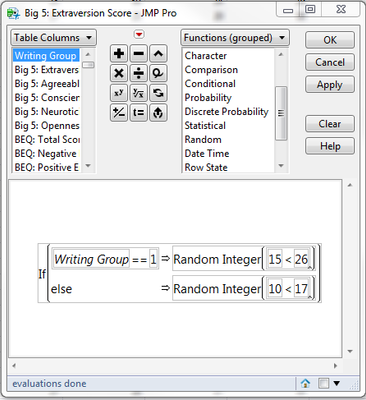Choose Language Hide Translation Bar
Highlighted

## If/Random Int Formula Question

Hello,

I am trying to get a formula to work for a dataset, but I'm having problems. I have the formula set up so that:But this returns 1 for all columns. What am I doing wrong?

3 REPLIES 3
Highlighted

## Re: If/Random Int Formula Question

Hi, Benson!

Try a Comma(,) instead of the LessThan(<) symbol in the Random, Integer function.

Alternatively, try not holding down the Shift key when you type the Comma.

Highlighted

## Re: If/Random Int Formula Question

Try replacing the less than sign with a comma   (15,26)

Highlighted

## Re: If/Random Int Formula Question

Try a comma instead of the less-than symbol between the numbers. The above returns a random integer between 1 and 1 (because 15 is less than 26. Every time)

To enable the optional second argument in the formula editor, select the square within parentheses and click ^.

Article Labels

There are no labels assigned to this post.